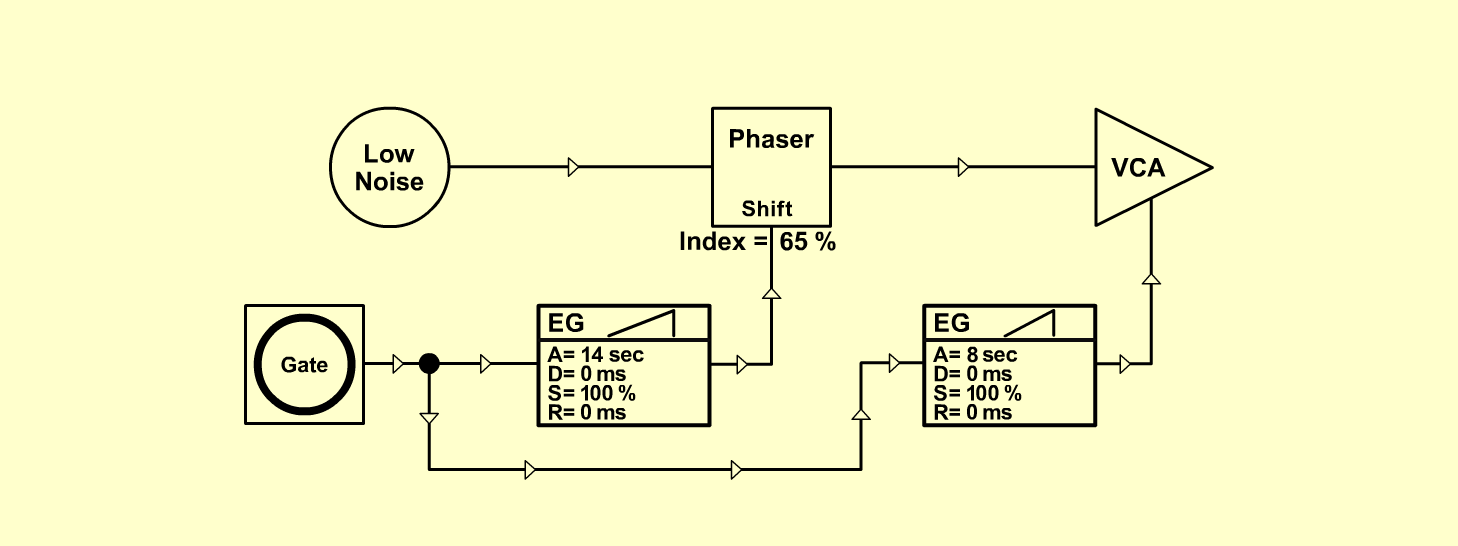Spaceship04FXD o e p f e r A - 100 Connections: Settings: A-118 (Colored) <=> A-125 (Audio In) A-140/1 (Output) <=> A-125 (CV)I A-140/2 (Output) <=> A-131 (CV1)I A-125 (Audio Out) <=> A-131 (Audio In)I A-118 (Blue = 0, Red = 10) A-140/1 (A = 5, D = 10, S = 10, R = 0, Range = H) A-140/2 (A = 3, D = 10, S = 10, R = 0, Range = H) A-125 (Level = 7, Shift = 0, CV = 6.5, Res = 10, Mix = 5) Notes: Accelerating spaceship Josef MuellerSound sample Spaceship04